# GED Math: Equations, Inequalities & Functions Chapter Exam

Exam Instructions:

Choose your answers to the questions and click 'Next' to see the next set of questions. You can skip questions if you would like and come back to them later with the yellow "Go To First Skipped Question" button. When you have completed the practice exam, a green submit button will appear. Click it to see your results. Good luck!

### Page 1

#### Question 1 1. What is the equation of the graph below?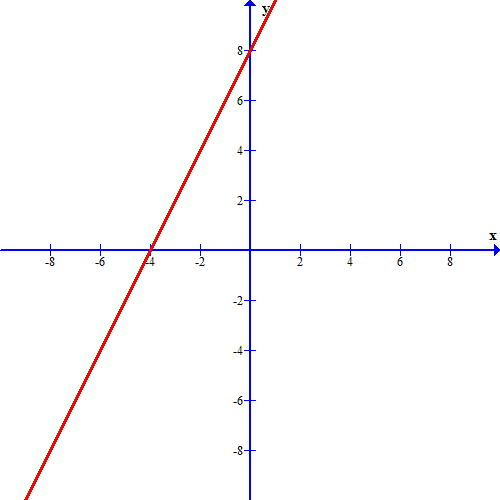### Page 3

#### Question 14 14. What is the solution to this graph of a linear system?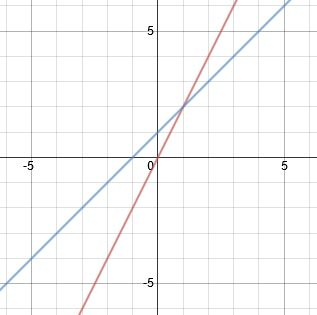### Page 4

#### Question 16 16. What is the b-value of the quadratic equation below?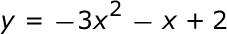#### Question 20 20. Use the quadratic formula to find the solutions to the equation below.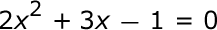### Page 5

#### Question 23 23. Which linear equation is shown below?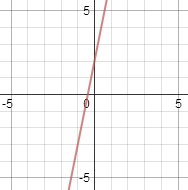#### Question 24 24. If the equation below told you a ball was 'h' feet in the air after 't' seconds, what would be the first step to finding out when it hit the ground?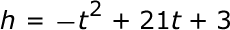### Page 6

#### Question 27 27. Find the roots of the equation below.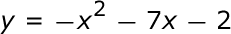#### Question 30 30. Which symbol is this?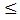#### GED Math: Equations, Inequalities & Functions Chapter Exam Instructions

Choose your answers to the questions and click 'Next' to see the next set of questions. You can skip questions if you would like and come back to them later with the yellow "Go To First Skipped Question" button. When you have completed the practice exam, a green submit button will appear. Click it to see your results. Good luck!

Support